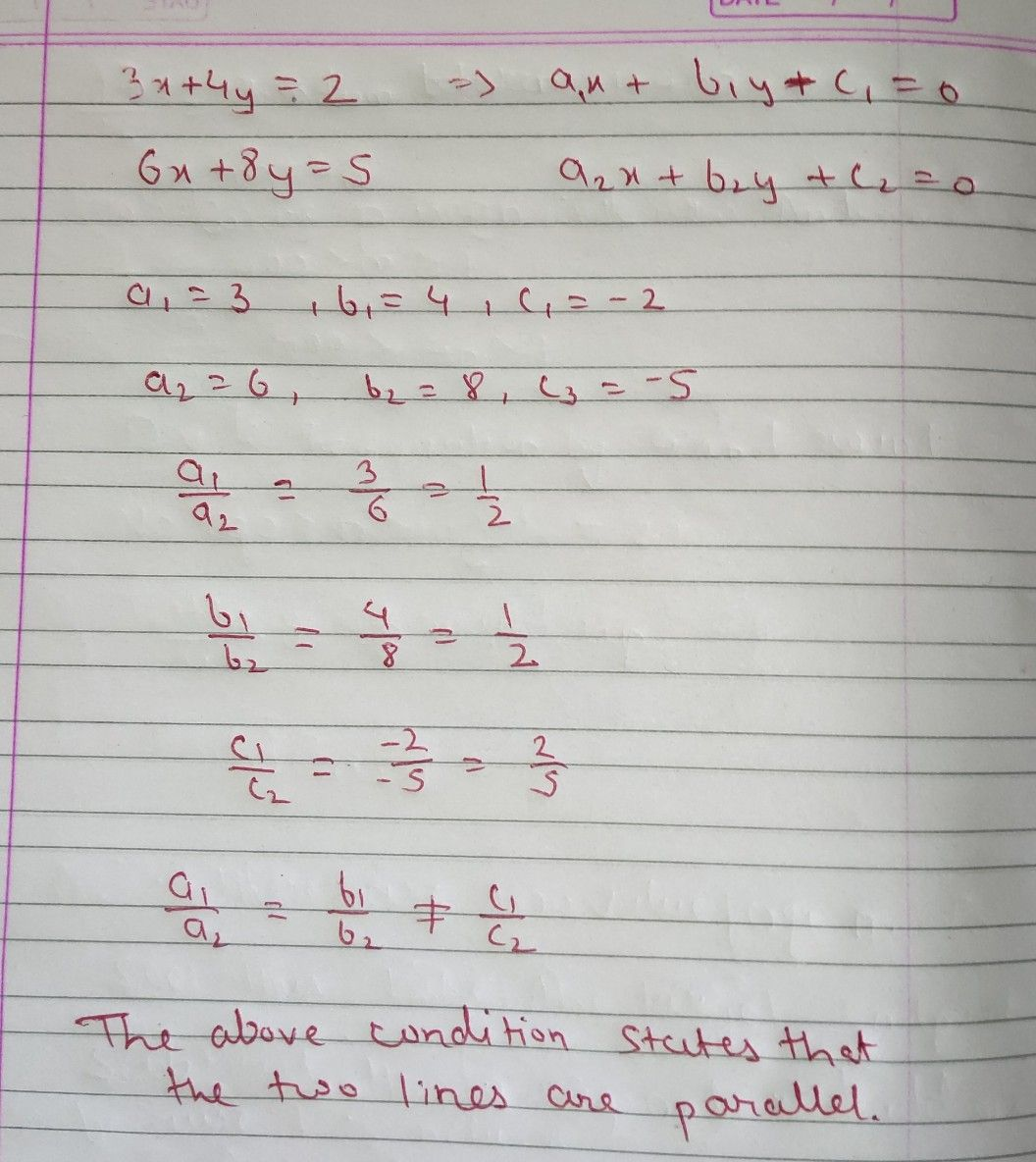Symbol
Problem$14$ Check whether the pair of linear equations $3x+4y=2and$ $6x+8y=5$ CHS) parallel, or coincident lines? $=$
10th-13th grade
Other
Search count: 120
SolutionQanda teacher - Zeus